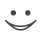Get these flashcards, study & pass exams. For free! Even on iPhone/Android!

Algebra Terms (19 Cards)

Expression
a term or terms that are connected by either addition or subtraction; Simplify or Evaluate
Equation
statements of equality between at least two terms; Solve
Inequalities
are statements that have either > or < between at least two terms
Variables
letters or symbols used to represent numbers or an unknown
Constants
specific numbers that are not multiplied by any variables
Coefficients
numbers that are multiplied by one or more variables
Terms
constant or variable expressions; separated only by addition and subtraction
Like or Similar Terms
terms that have the same variables raised to the same power
Substitution
replacing a variable with a known quantity(number)
Combining Like Terms
adding or subtracting terms that have the same variables raised to the same power; this method is used to simplify expressions
Order of Operations
1. Parenthesis and other grouping devices
2. Exponents and Square Roots
3. Multiplication and/or Division from Left to Right
4. Addition and/or Subtraction from Left to Right
Isolate the Variables
this is the goal when trying to solve an equation
Inverse Operations
Operations that “undo” one another; Addition/Subtraction, Multiplication/Division, Powers/Square Roots
whatever is added/subtracted from one side of an equation must be added/subtracted from the other side of the equation
Multiplication/Division Property of Equality
whatever is multiplied/divided from one side of an equation must be multiplied/divided from the other side of the equation
a number that when added to the original given number yields(equals) zero
Multiplicative Inverse
a number that when multiplied by the original given number yields(equals) one
any number(n) plus zero is equal to n
Multiplicative Identity
any number(n) multiplied by one is equal to nFlashcard set info:
Author: CoboCards-User
Main topic: Algebra Vocabulary and Properties
Topic: Math
School / Univ.: Garfield Middle School
City: Lakewood
Published: 18.02.2015
Tags: Algebra

Card tags:
All cards (19)
no tagsReport abuse